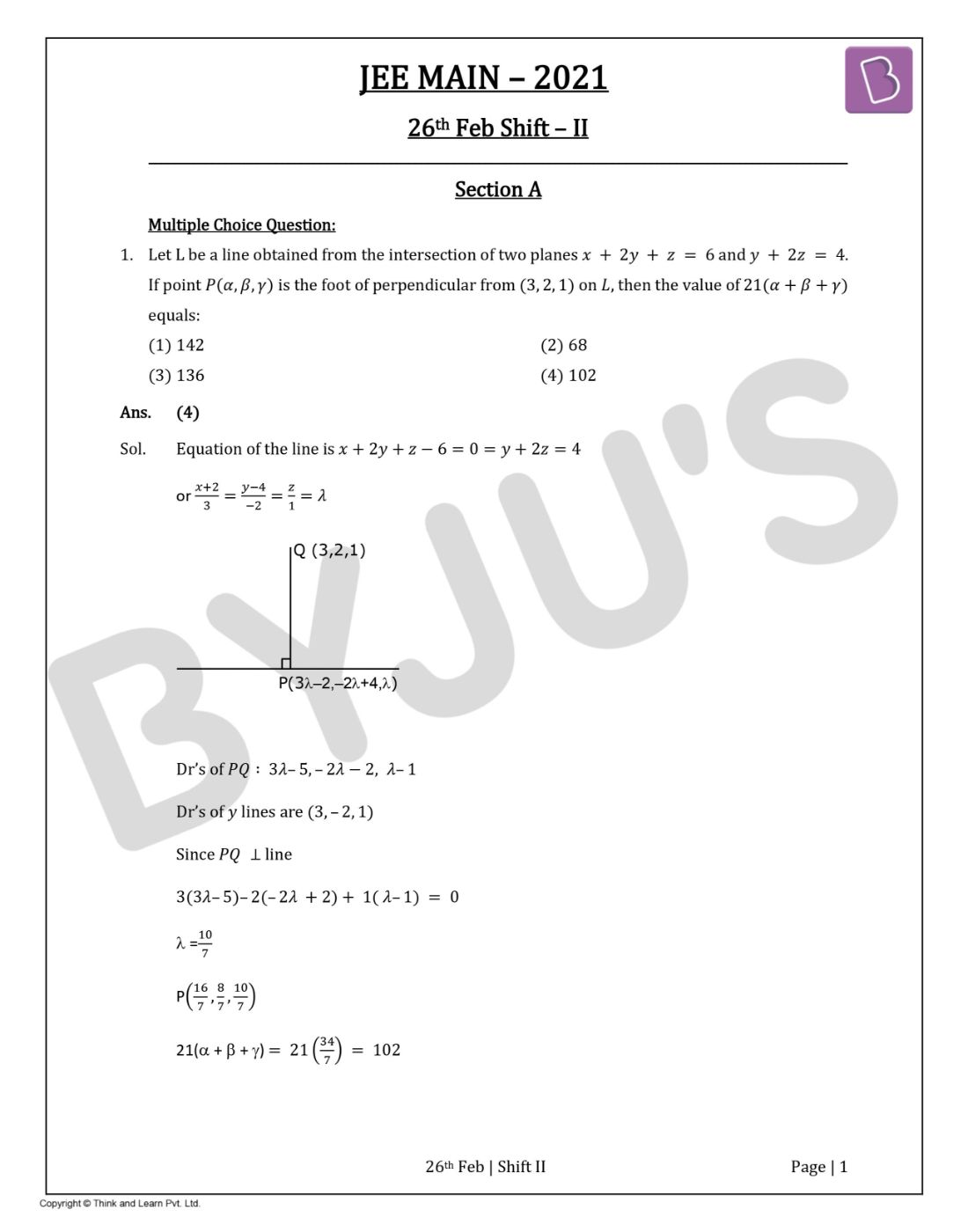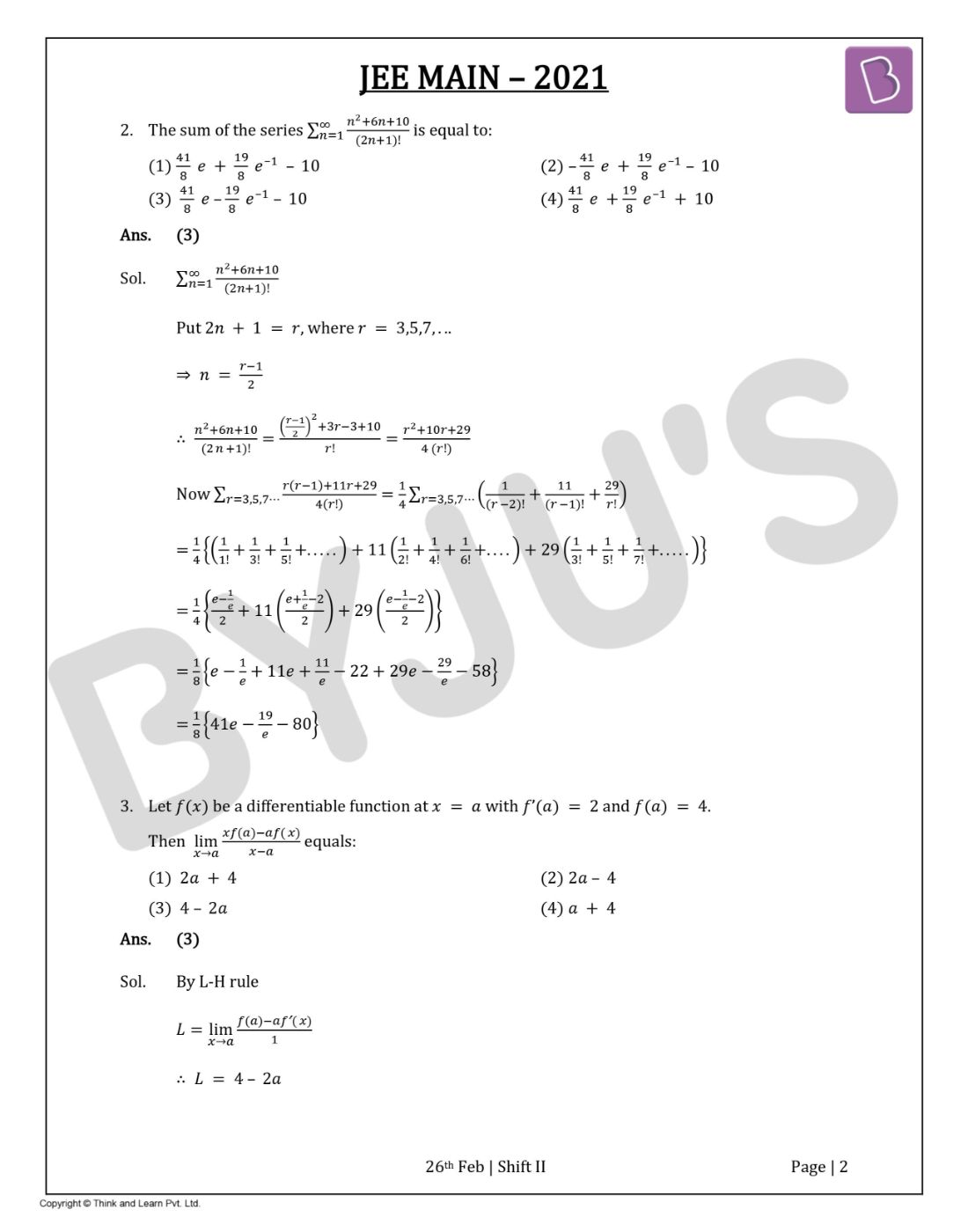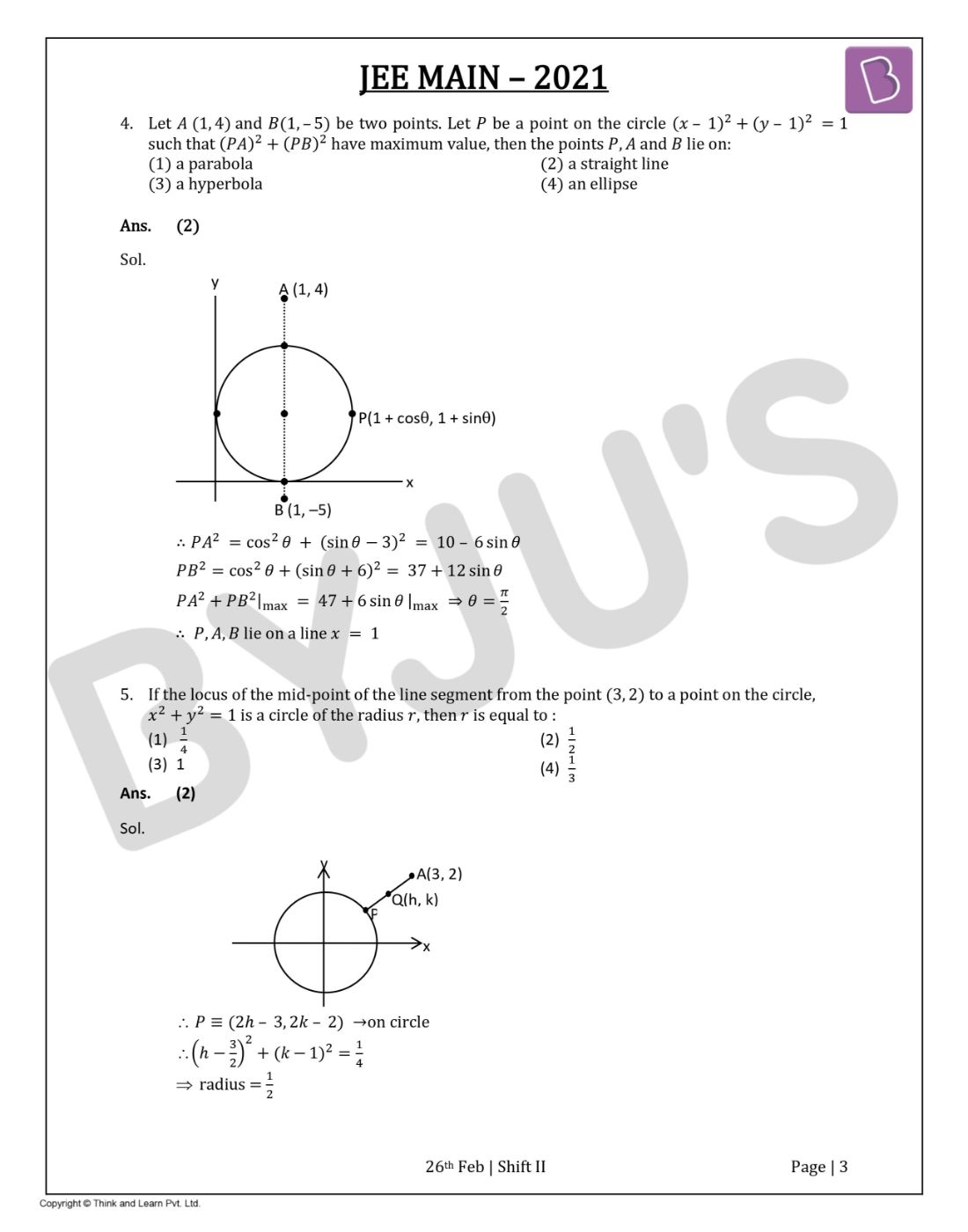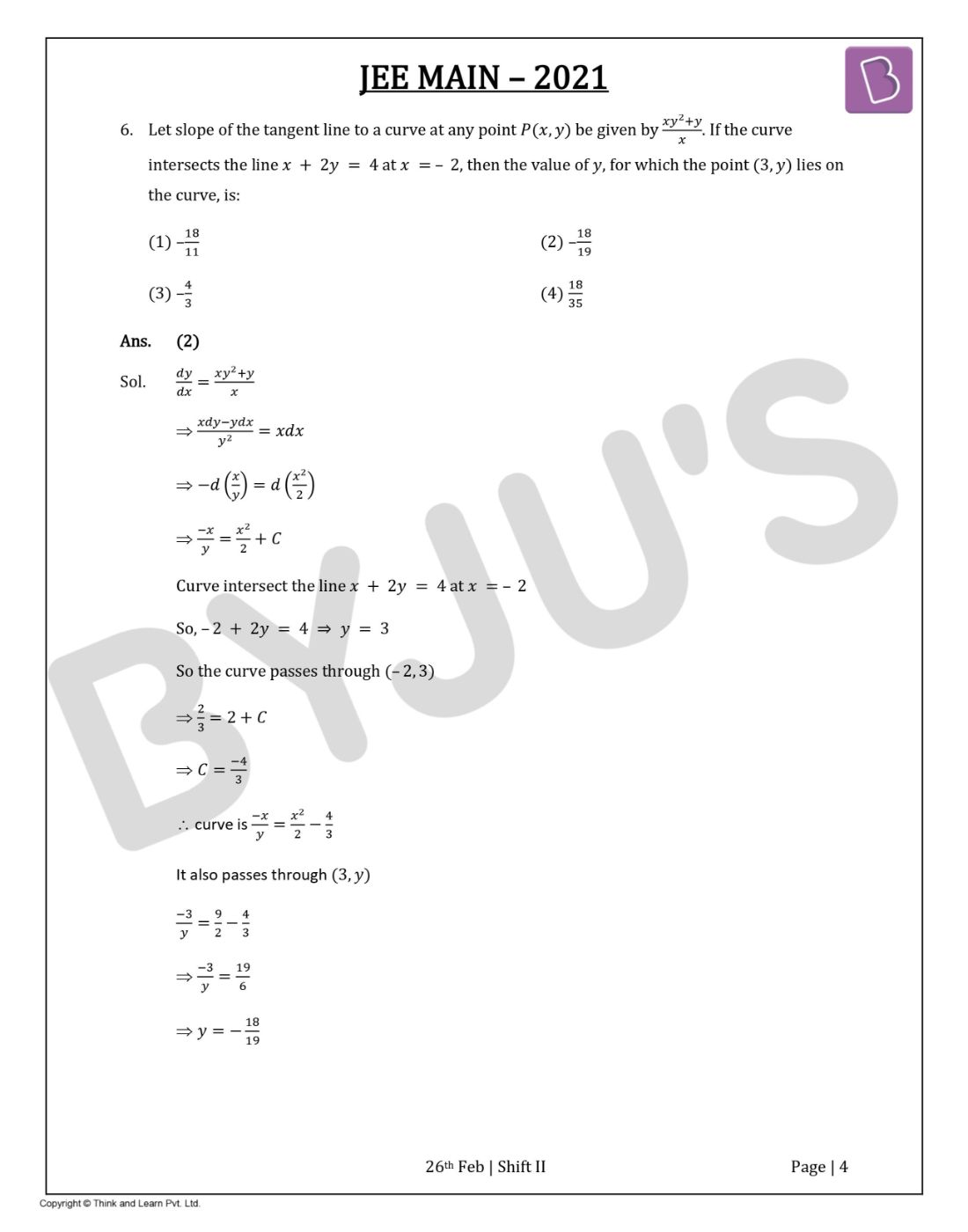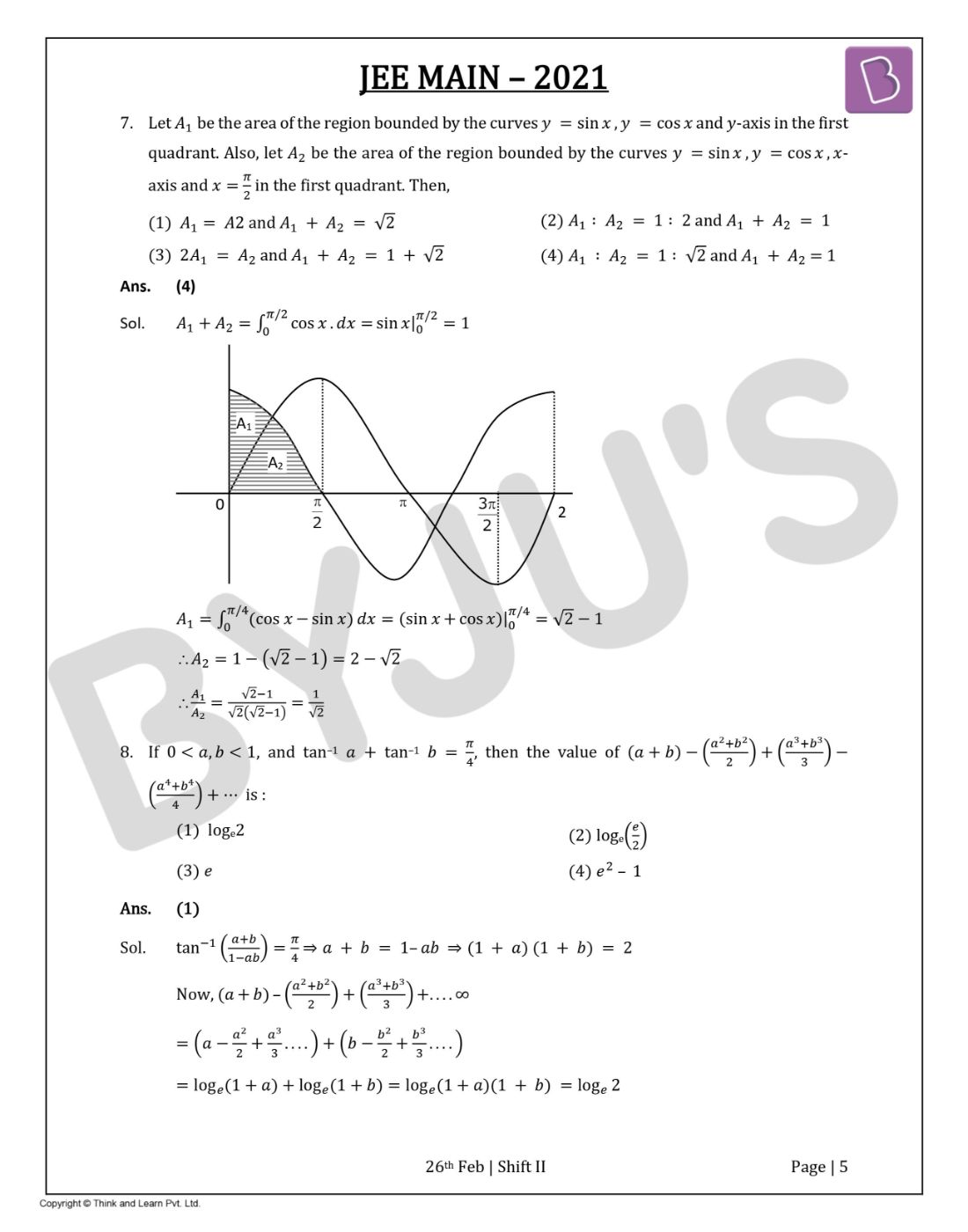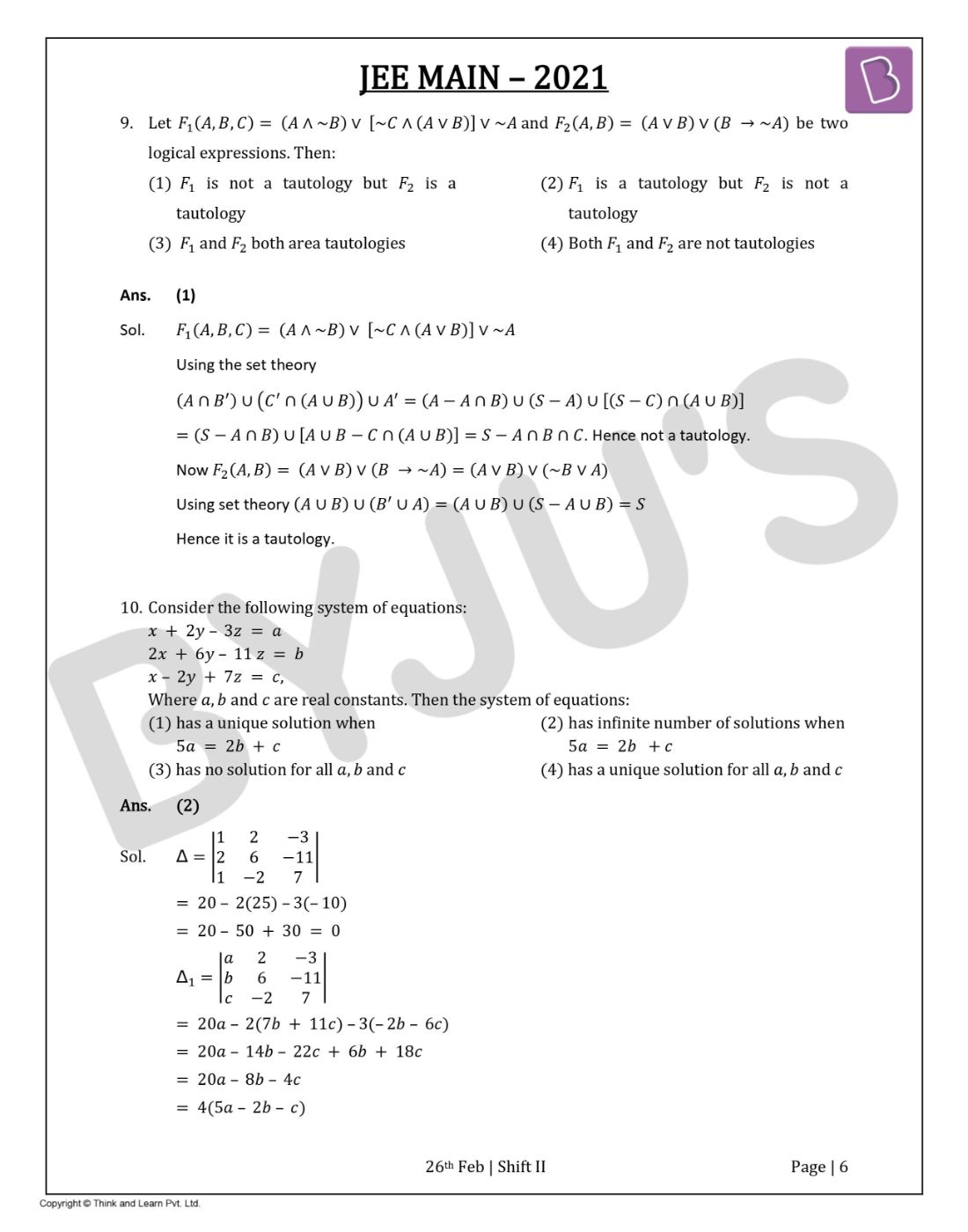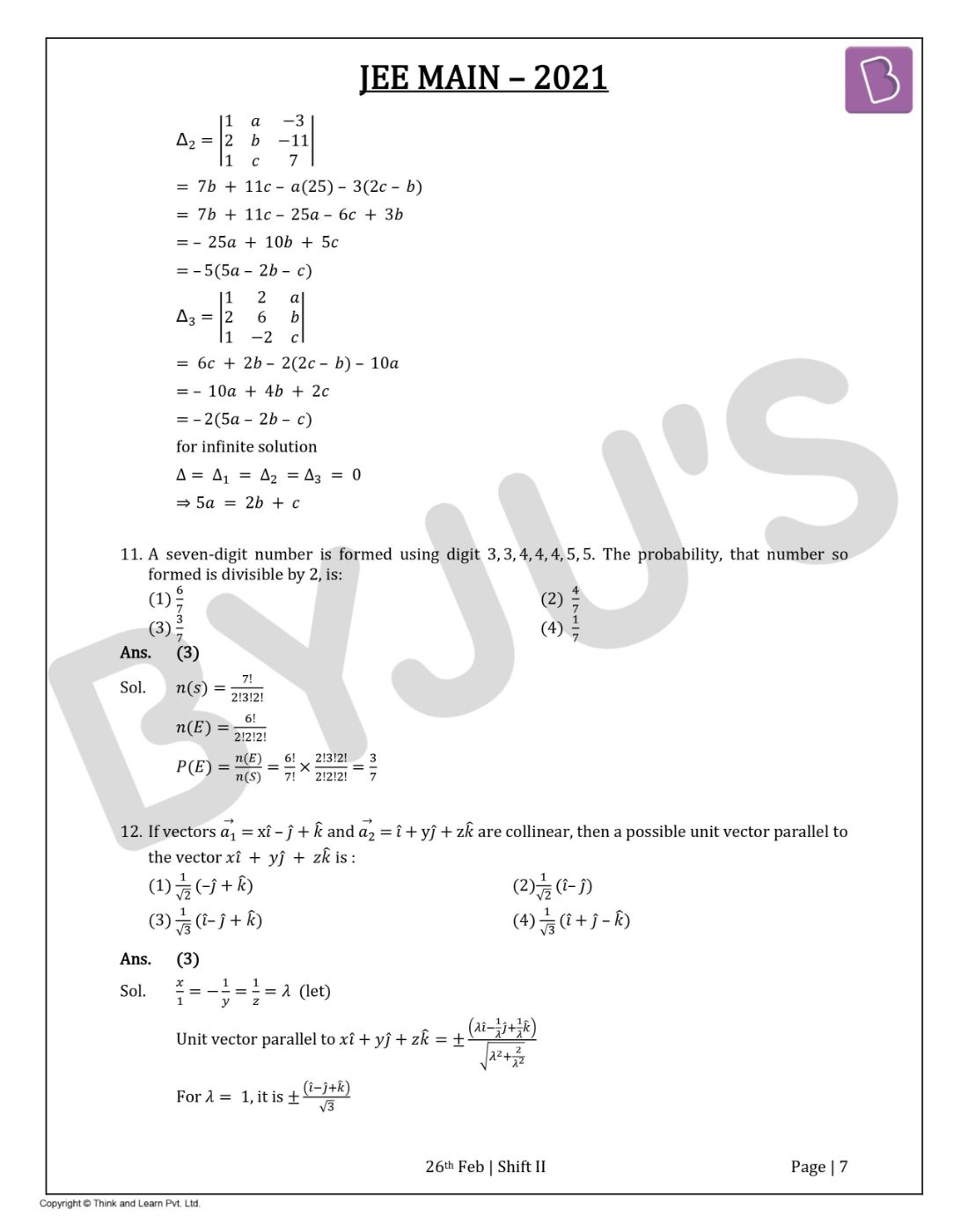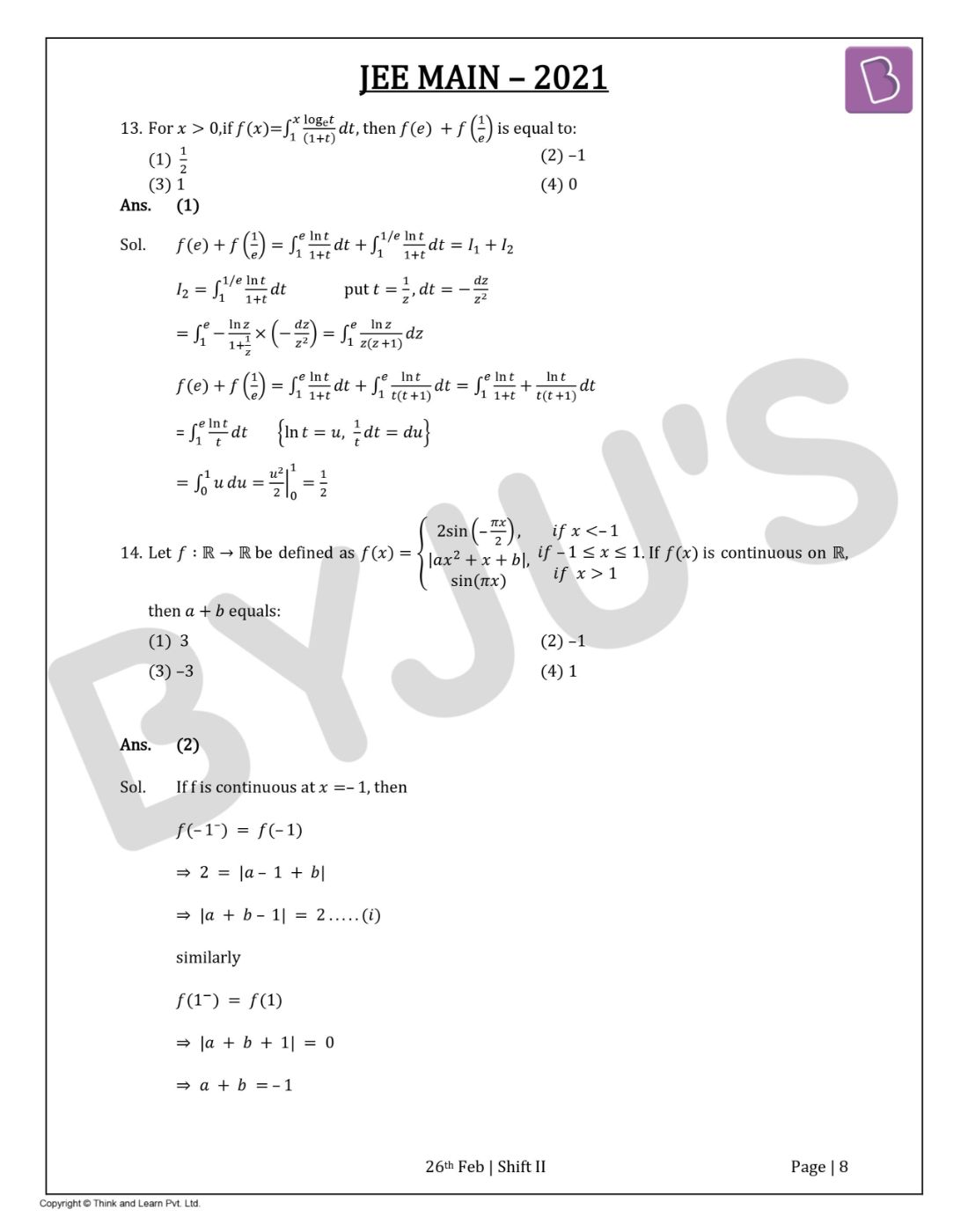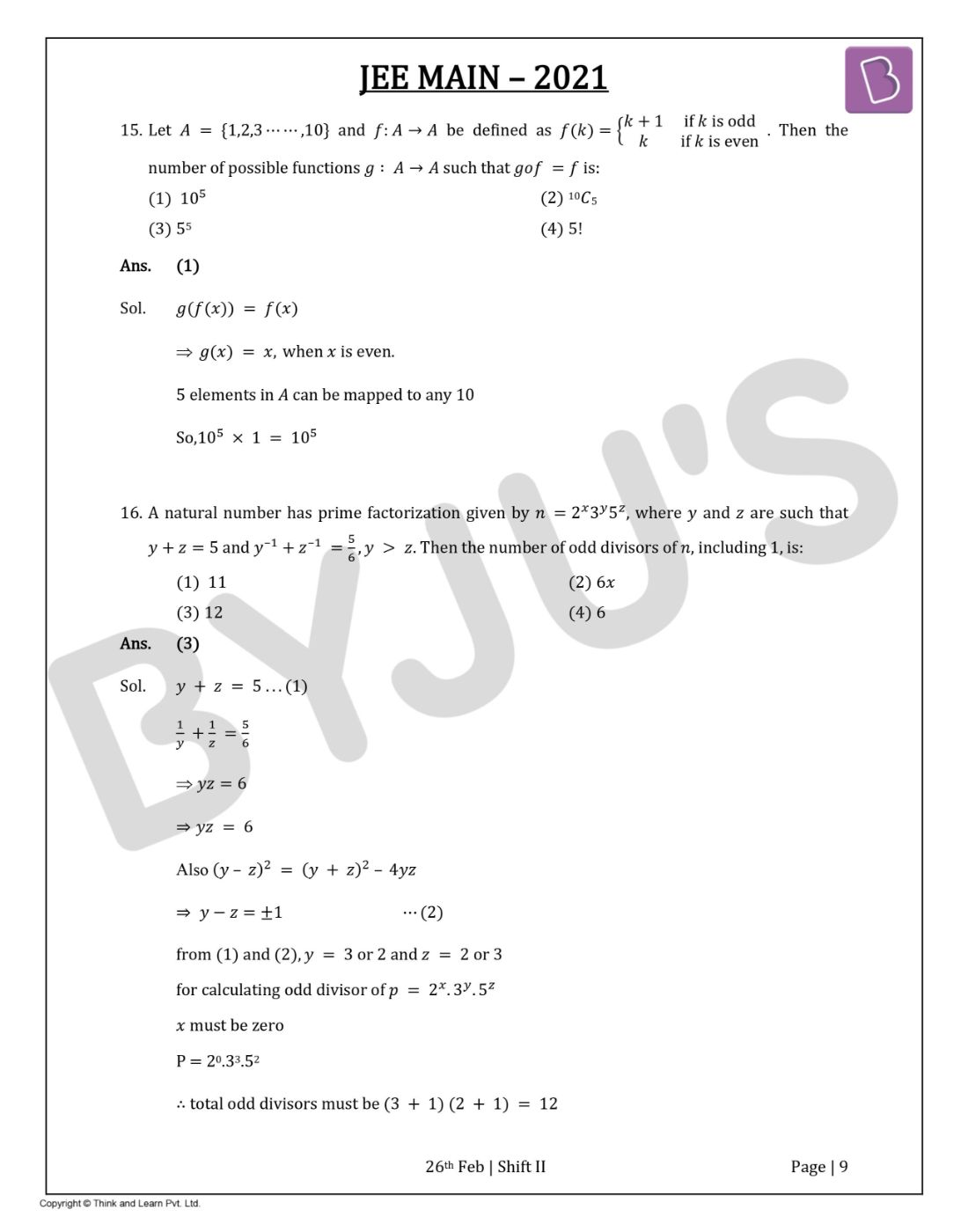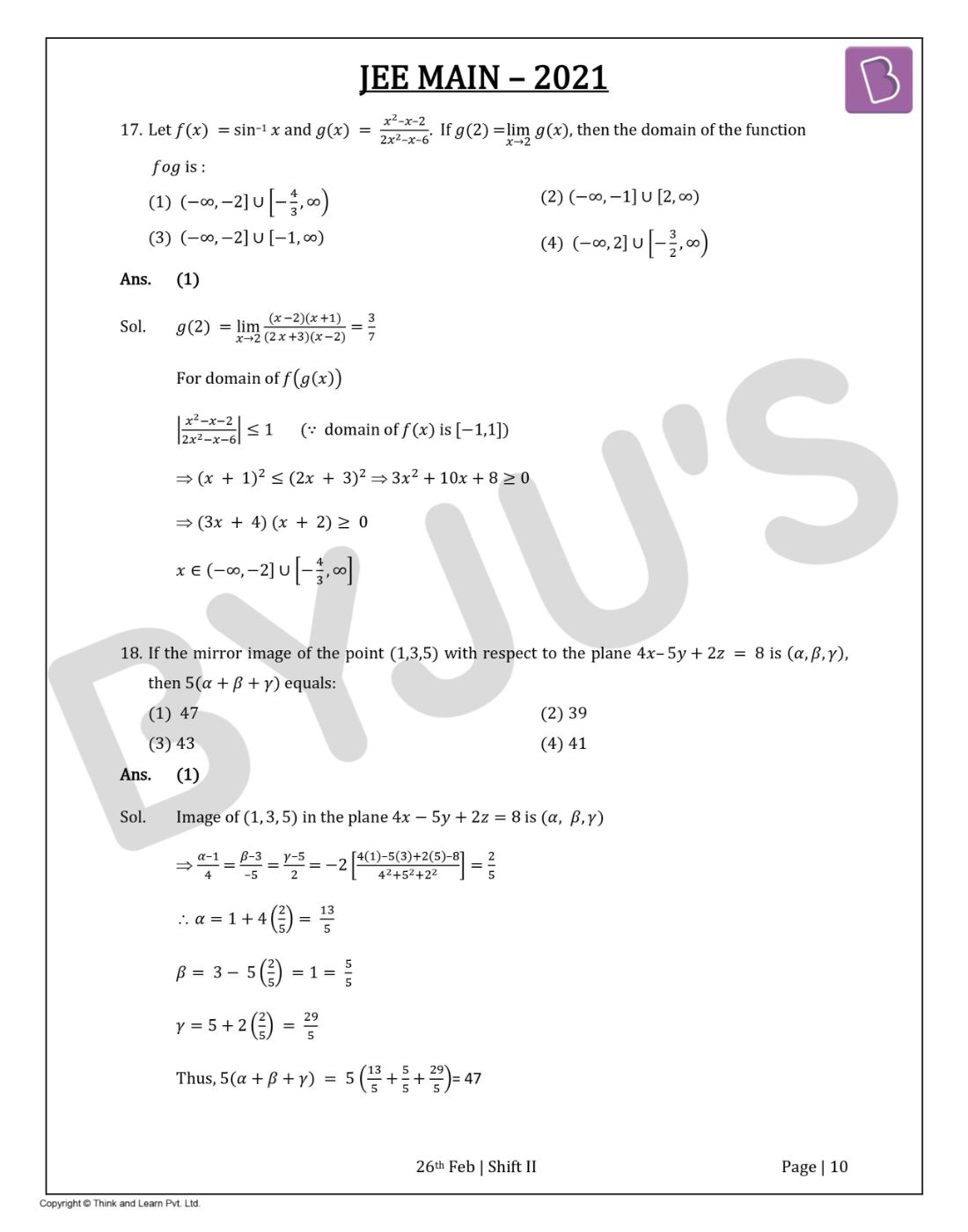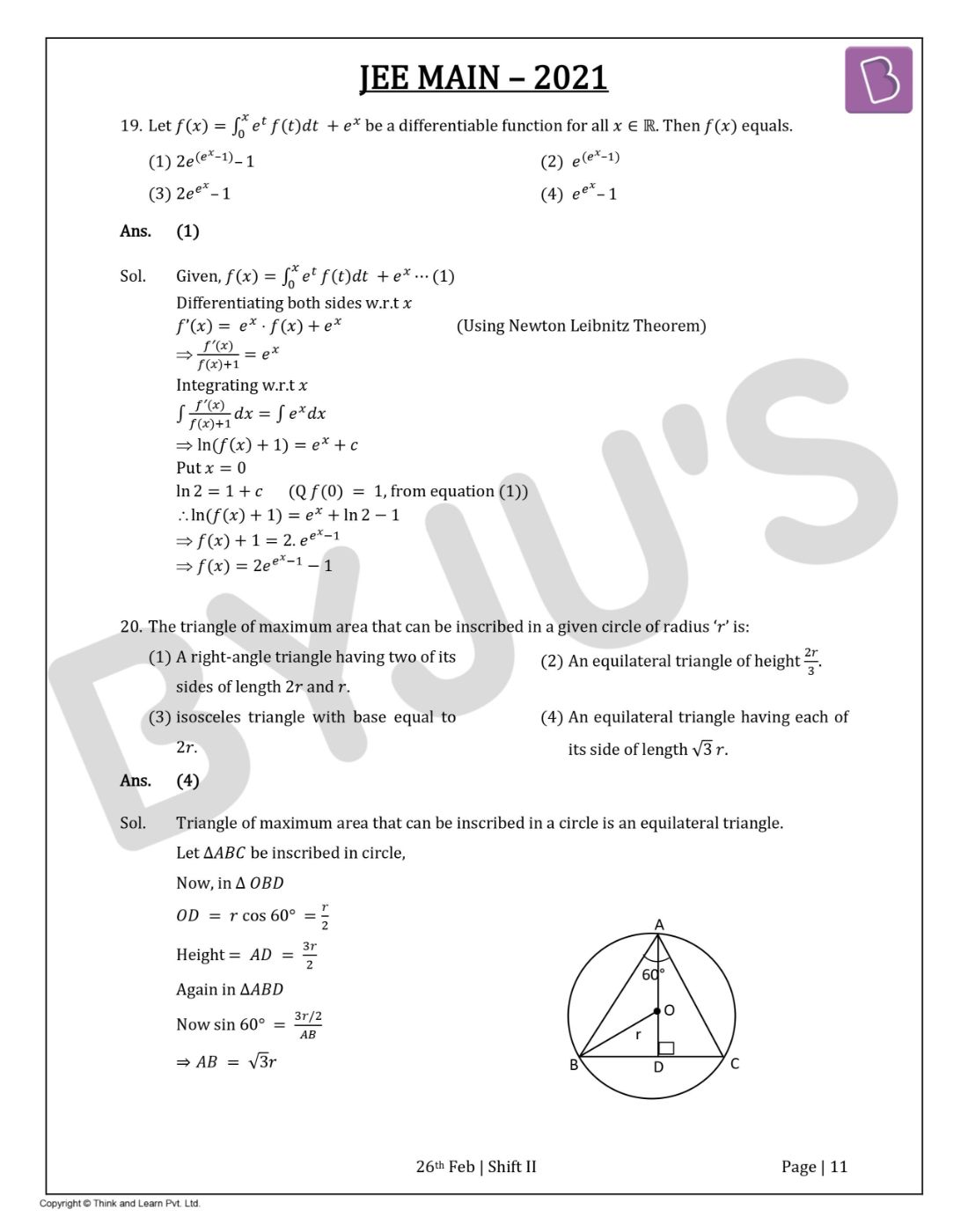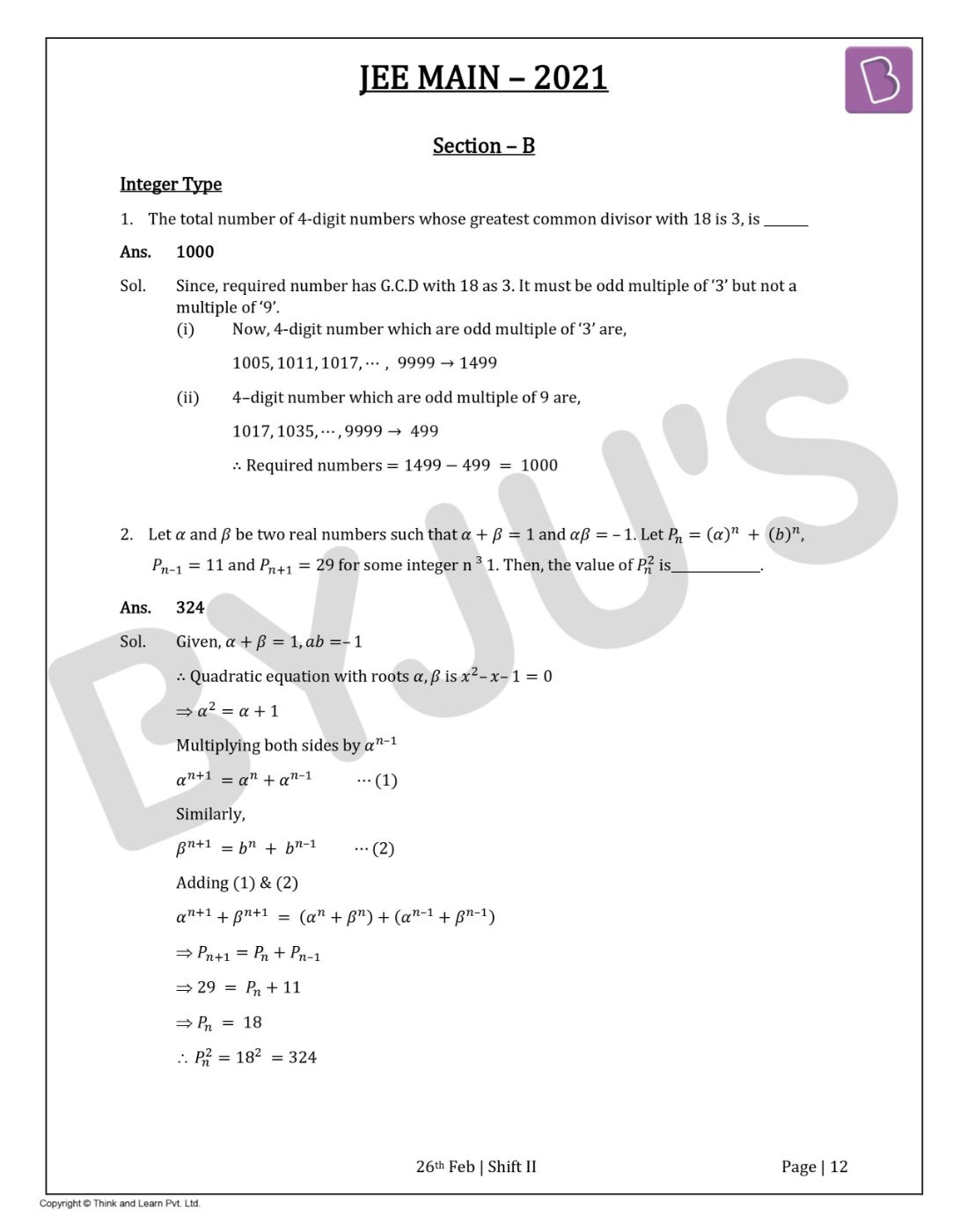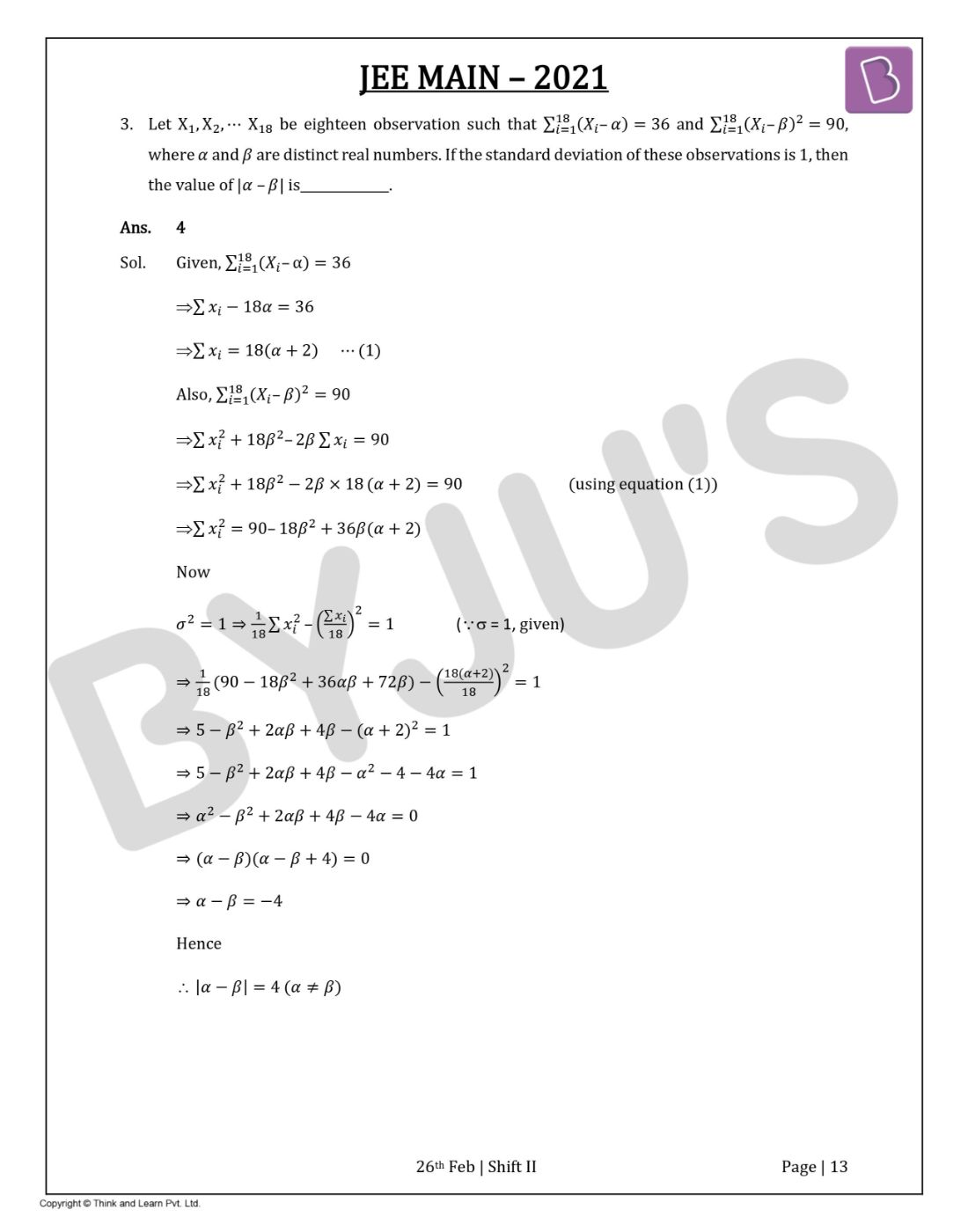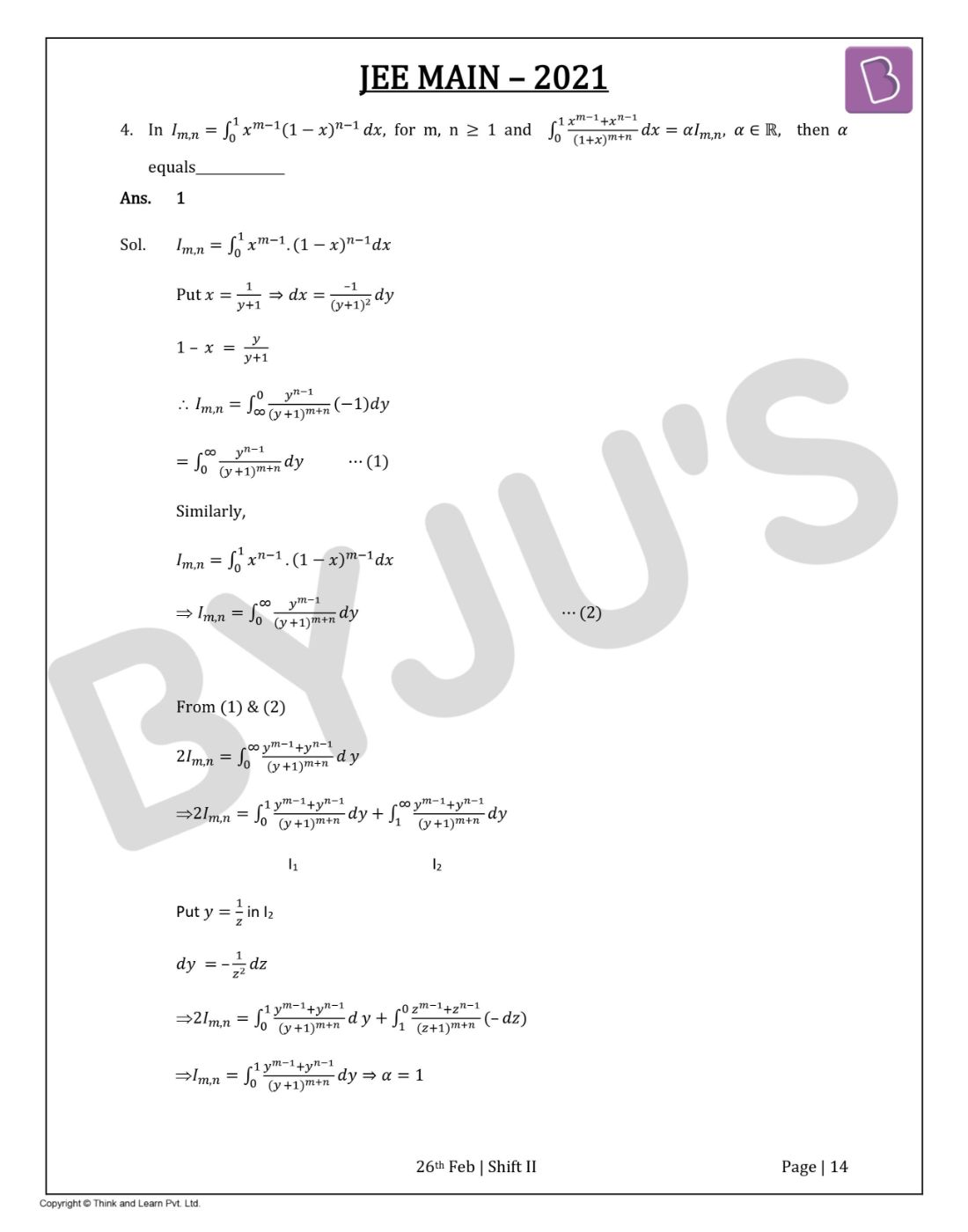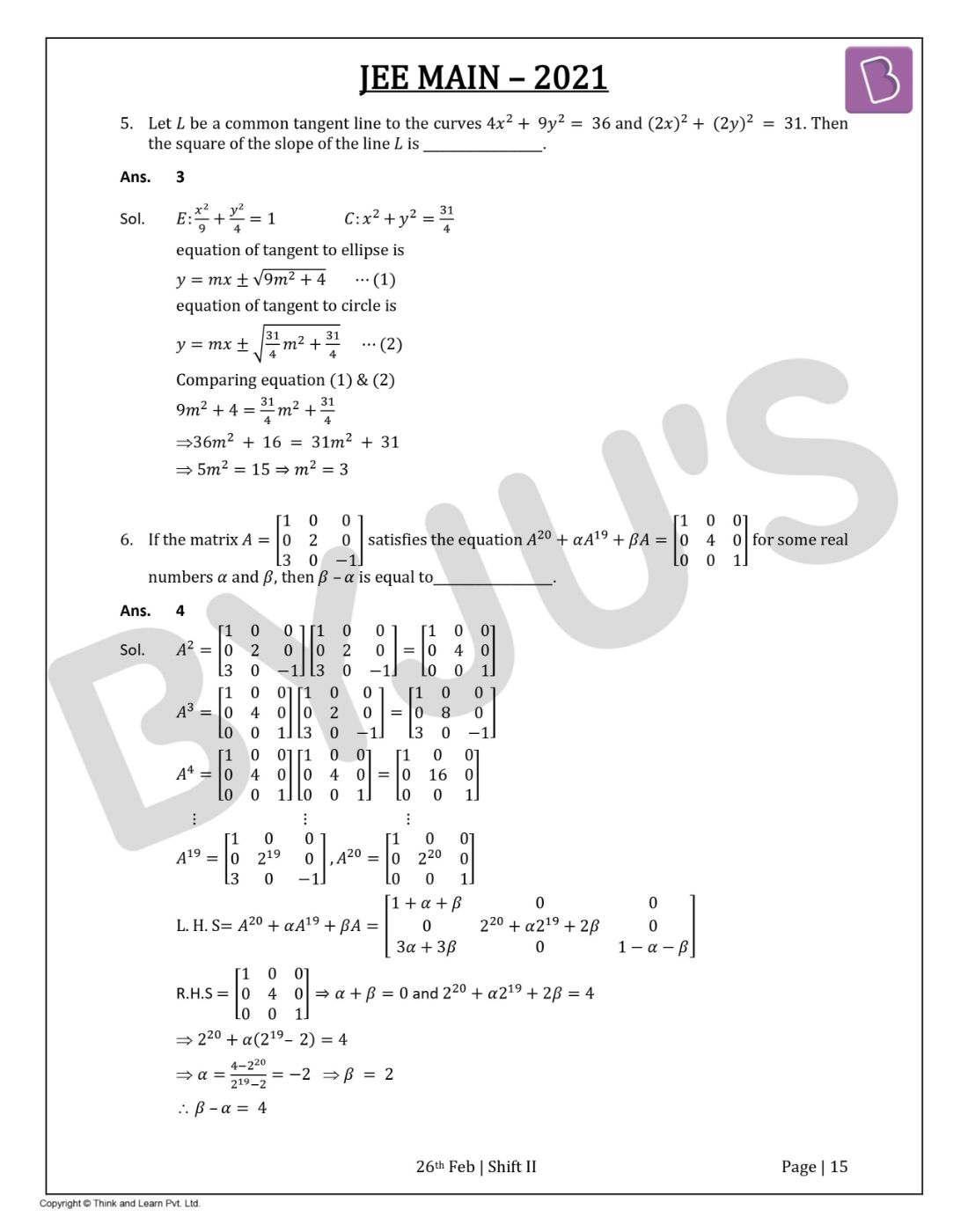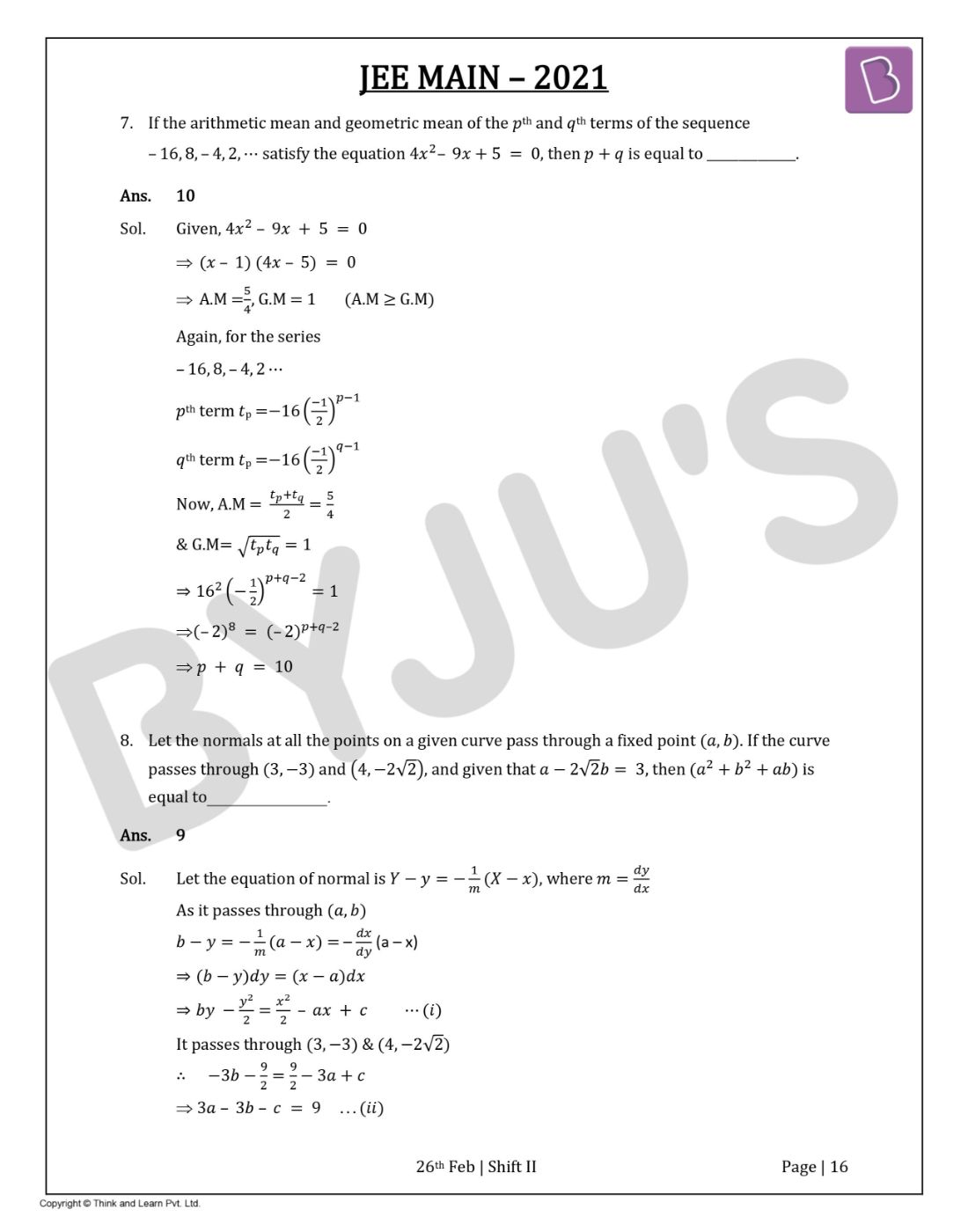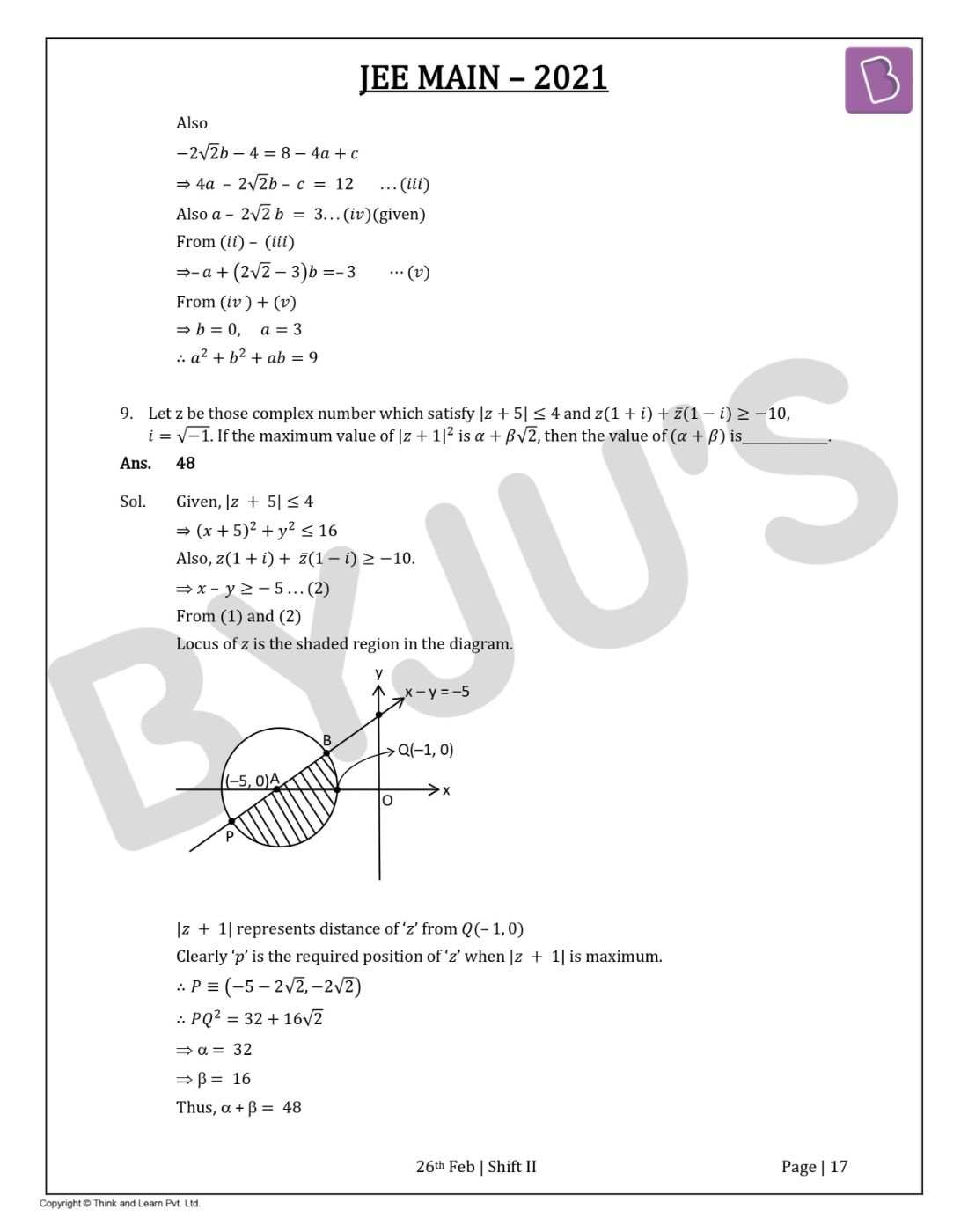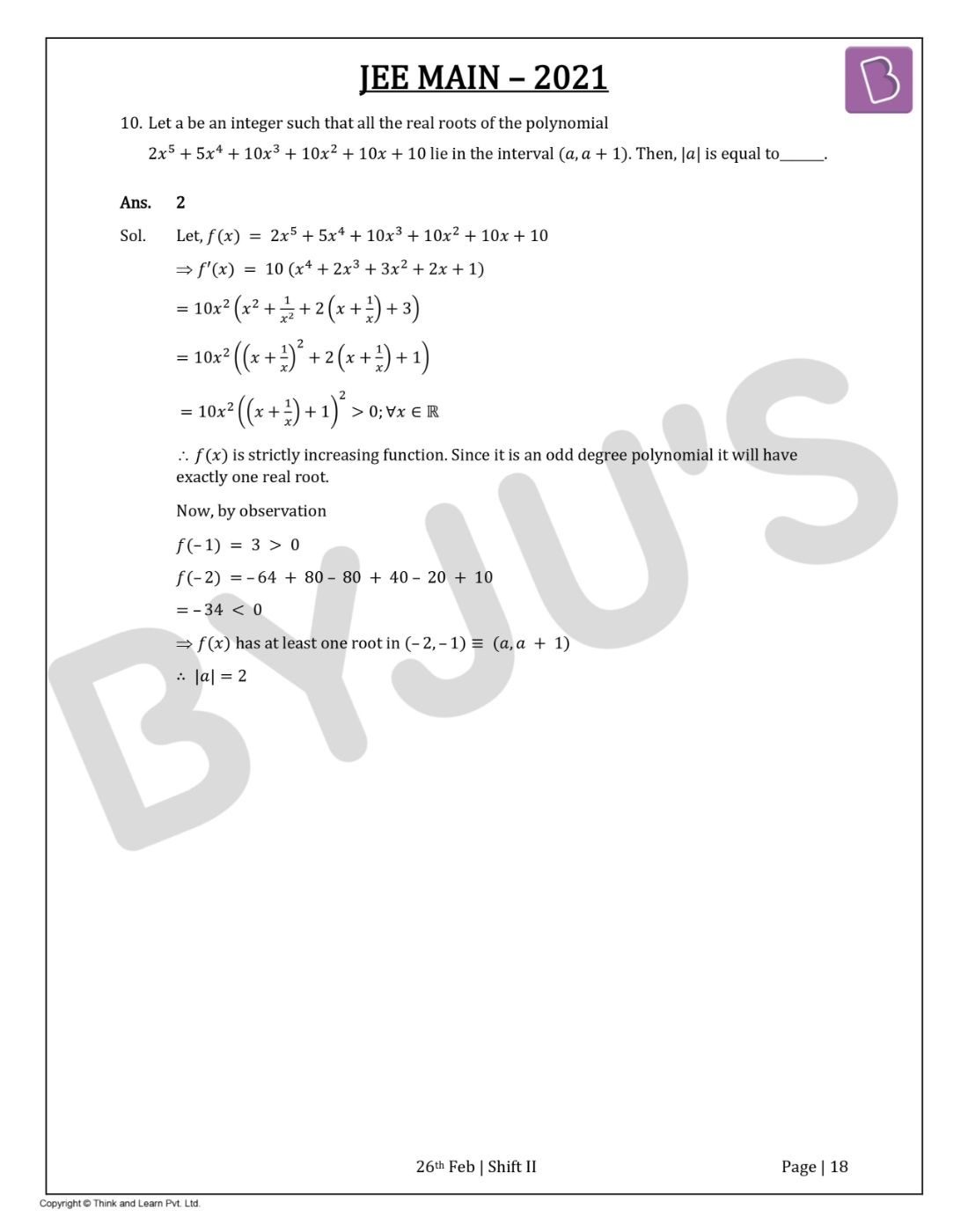Win up to 100% scholarship on Aakash BYJU'S JEE/NEET courses with ABNAT Win up to 100% scholarship on Aakash BYJU'S JEE/NEET courses with ABNAT

# JEE Main 2021 February 26 – Shift 2 Maths Question Paper with Solutions

JEE aspirants will find solutions for all the questions asked in JEE Main 2021 Mathematics paper for February 26th Shift 2 exam on this page. The set of solutions have been prepared by BYJU’s expert teachers. The solutions have been crafted in a detailed manner. Practising these questions will help every JEE aspirant to understand the overall exam pattern and prepare accordingly.

JEE Main 2021 26th February Shift 2 Maths Question Paper

SECTION A

Question 1: Let L be a line obtained from the intersection of two planes x + 2y + z = 6 and y + 2z = 4. If point P (?, β, γ) is the foot of the perpendicular from (3, 2, 1) on L, then the value of 21 (? + β + γ) equals:

a. 142
b. 68
c. 136
d. 102

Equation of the line is x + 2y + z – 6 = 0 = y + 2z = 4

[x + 2] / 3 = [y – 4] / – 2 = z / 1 = λ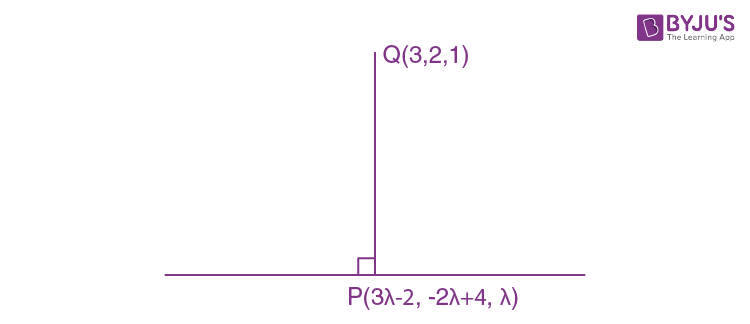Dr’s of PQ: 3λ – 5, – 2λ – 2, λ – 1

Dr’s of y lines are (3, – 2, 1)

Since PQ is perpendicular to the line.

3 (3λ – 5) – 2 (- 2λ + 2) + 1 (λ – 1) = 0

λ = 10 / 7

P (16 / 7, 8 / 7, 10 / 7)

21 (? + β + γ) = 21 (34 / 7) = 102

Question 2: The sum of the series ∑n=1∞[n2 + 6n + 10] / (2n + 1)! is equal to:

a. (41/ 8) e + (19 / 8) e-1 – 10
b. (- 41/ 8) e + (19 / 8) e-1 – 10
c. (41/ 8) e – (19 / 8) e-1 – 10
d. (41/ 8) e + (19 / 8) e-1 + 10

n=1∞[n2 + 6n + 10] / (2n + 1)!

Put 2n + 1 = r, where r = 3, 5, 7…..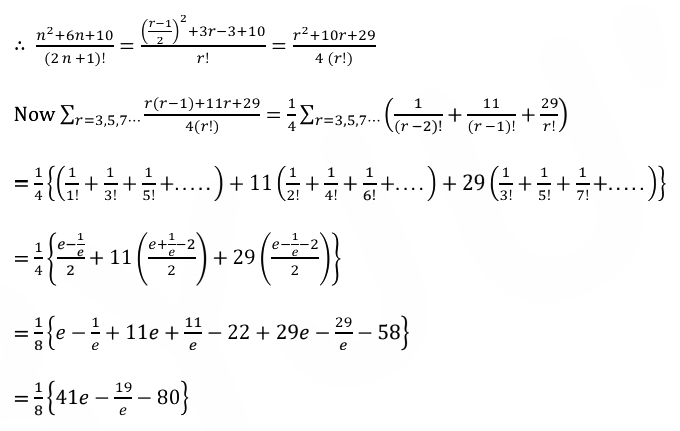n = [r – 1] / 2

Question 3: Let f(x) be a differentiable function at x = a with f’ (a) = 2 and f (a) = 4. Then limx→a [x f (a) – a f (x)] / [x – a] equals:

a. 2a + 4
b. 2a – 4
c. 4 – 2a
d. a + 4

By L-H rule

L = limx→a [f (a) – a f’ (x)] / 

L = 4 – 2a

Question 4: Let A (1, 4) and B (1, – 5) be two points. Let P be a point on the circle (x – 1)2 + (y – 1)2 = 1 such that (PA)2 + (PB)2 have maximum value, then the points P, A and B lie on:

a. a parabola
b. a straight line
c. a hyperbola
d. an ellipse

PA2 = cos2 θ + (sin θ – 3)2 = 10 – 6 sin θ

PB2 = cos2 θ + (sin θ + 6)2 = 37 + 12 sin θ

PA2 + PB2|max = 47 + 6 sin θ|max

θ = π / 2

The points P, A and B lie on the line x = 1.

Question 5: If the locus of the mid-point of the line segment from the point (3, 2) to a point on the circle, x2 + y2 = 1 is a circle of the radius r, then r is equal to :

a. 14
b. 12
c. 1
d. 13

P ≡ (2h – 3), (2k – 2) → on circle

[h – (3 / 2)]2 + (k – 1)2 = 1 / 4

Question 6: Let the slope of the tangent line to a curve at any point P (x, y) be given by [xy2 + y] / x. If the curve intersects the line x + 2y = 4 at x = – 2, then the value of y, for which the point (3, y) lies on the curve, is:

a. – 18 / 11
b. – 18 / 19
c. – 4 / 3
d. 18 / 35

dy / dx = [xy2 + y] / x

[x dy – y dx] / y2 = x dx

– d (x / y) = d (x2 / 2)

(- x / y) = (x2 / 2) + c

The curve intersects the line x + 2y = 4 at x = – 2.

So, – 2 + 2y = 4

y = 3

The curve passes through (-2, 3).

(2 / 3) = 2 + c

c = – 4 / 3

The curve is (- x / y) = (x2 / 2) – (4 / 3).

It also passes through (3, y).

(- 3 / y) = (9 / 2) – (4 / 3)

y = – 18 / 19

Question 7: Let A1 be the area of the region bounded by the curves y = sinx, y = cosx and y-axis in the first quadrant. Also, let A2 be the area of the region bounded by the curves y = sinx, y = cosx, the x-axis and x = π / 2 in the first quadrant. Then,

a. A1 = A2 and A1 + A2 = √2
b. A1 : A2 = 1 : 2 and A1 + A2 = 1
c. 2A1 = A2 and A1 + A2 = 1 + √2
d. A1 : A2 = 1 : √2 and A1 + A2 = 1

A1 + A2 = ∫0π/2 cosx dx = sin x|0π/2 = 1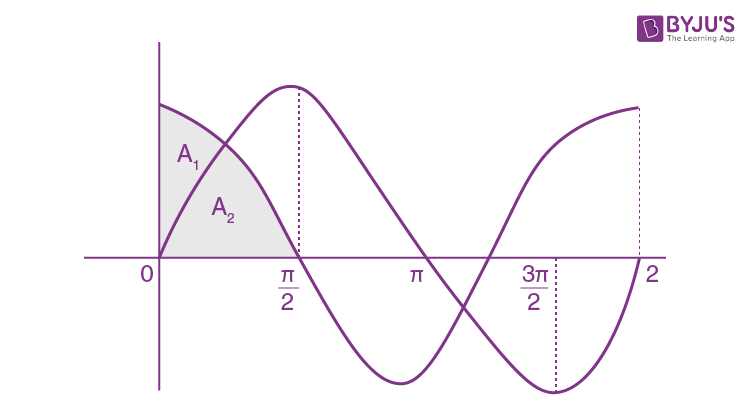A1 = ∫0π/4 (cosx – sinx) dx = (sinx + cosx)0π/4 = √2 – 1

A2 = 1 – (√2 – 1) = 2 – √2

A1 / A2 = (√2 – 1) / (√2 (√2 – 1)) = 1 / √2

Question 8: If 0 < a, b < 1, and tan-1 a + tan-1 b = π / 4, then the value of (a + b) – [a2 + b2] / 2 + [a3 + b3 ] / 3 – [a4 + b4] / 4 + …. is

a. loge2
b. loge (e / 2)
c. e
d. e2 – 1

tan-1 {[a + b] / [1 – ab]} = (π / 4)

a + b = 1 – ab

(1 + a) (1 + b) = 2

(a + b) – [a2 + b2] / 2 + [a3 + b3] / 3 +…infinity

= [a – (a2 / 2) + (a3 / 3) …..] + [b – (b2 / 2) + (b3 / 3) …..]

= loge (1 + a) + loge (1 + b)

= loge (1 + a) (1 + b) = loge 2

Question 9: Let F1 (A, B, C) = (A ∧ ~B) ∨ [~C ∧ (A ∨ B)] ∨ ~A and F2 (A, B) = (A ∨ B) ∨ (B → ~A) be two logical expressions. Then:

a. F1 is not a tautology but F2 is a tautology
b. F1 is a tautology but F2 is not a tautology
c. F1 and F2 both area tautologies
d. Both F1 and F2 are not tautologies

F1 (A, B, C) = (A ∧ ~B) ∨ [~C ∧ (A ∨ B)] ∨ ~A

Using the set theory

(A ∩ B′) ∪ (C′ ∩ (A ∪ B)) ∪ A′ = (A − A ∩ B) ∪ (S − A) ∪ [(S − C) ∩ (A ∪ B)]

= (S − A ∩ B) ∪ [A ∪ B − C ∩ (A ∪ B)]

= S − A ∩ B ∩ C.

Hence not a tautology.

Now F2 (A, B) = (A ∨ B) ∨ (B → ~A) = (A ∨ B) ∨ (~B ∨ A)

Using set theory (A ∪ B) ∪ (B′ ∪ A) = (A ∪ B) ∪ (S − A ∪ B) = S

Hence it is a tautology.

Question 10: Consider the following system of equations:

x + 2y – 3z = a

2x + 6y – 11 z = b

x – 2y + 7z = c,

Where a, b and c are real constants. Then the system of equations:

a. has a unique solution when 5a = 2b + c
b. has an infinite number of solutions when 5a = 2b + c
c. has no solution for all a, b and c
d. has a unique solution for all a, b and c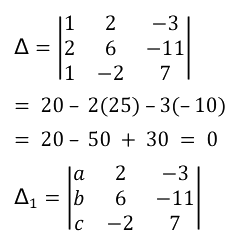= 20a – 2(7b + 11c) – 3(- 2b – 6c)

= 20a – 14b – 22c + 6b + 18c

= 20a – 8b – 4c

= 4(5a – 2b – c)

$$\begin{array}{l}\Delta _{2} = \begin{vmatrix} 1 & a & -3 \\ 2& b & -11\\ 1& c & 7 \end{vmatrix}\end{array}$$

= 7b+ 11c – a(25) – 3(2c – b)

= 7b+ 11c – 25a – 6c + 3b

= – 25a + 10b+ 5c

= – 5(5a – 2b- c)

$$\begin{array}{l}\Delta _{3} = \begin{vmatrix} 1 & 2 & a\\ 2& 6 & b\\ 1& -2 & c \end{vmatrix}\end{array}$$

= 6c + 2b- 2(2c – b) – 10a

= – 10a + 4b+ 2c

= – 2(5a – 2b- c)

For infinite solution,

Δ = Δ1 = Δ2 = Δ3 = 0

⇒ 5a = 2b + c

Question 11: A seven-digit number is formed using the digit 3, 3, 4, 4, 4, 5, 5. The probability, that number so formed is divisible by 2, is:

a. 6 / 7
b. 4 / 7
c. 3 / 7
d. 1 / 7

n (S) = 7! / [2! 3! 2!]

n (E) = 6! / [2! 2! 2!]

P (E) = n (E) / n (S) = [6! / 7!] * [[2! 3! 2!] / [2! 2! 2!]] = 3 / 7

Question 12: If vectors a1 = xi – j + k and a2 = i + yj + zk are collinear, then a possible unit vector parallel to the vector xi + yj + zk is :

a. (1 / √2) (- j + k)
b. (1 / √2) (i – j)
c. (1 / √3) (i – j + k)
d. (1 / √3) (i + j – k)

(x / 1) = – 1 / y = 1 / z = λ (let)

Unit vector parallel to xi + yj + zk = ± [λi – (1 / λ) j + (1 / λ) k] / √λ2 + (2 / λ2)

For λ = 1, it is ± [i – j + k] / √3

Question 13: For x > 0, if f (x) = ∫1x loge t / [1 + t] dt, then f (e) + f (1 / e) is equal to:

a. 1 / 2
b. -1
c. 1
d. 0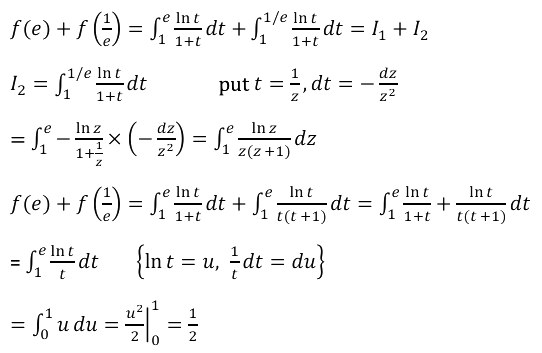Question 14: Let f : R → R be defined as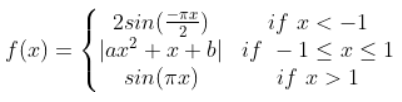If f (x) is continuous on R, then a + b equals:

a. 3
b. -1
c. -3
d. 1

If f is continuous at x = – 1, then f (- 1) = f (- 1)

2 = |a – 1 + b|

|a + b – 1| = 2 — (i)

Similarly f (1) = f (1)

|a + b + 1| = 0

a + b = – 1

Question 15: Let A = {1, 2, 3 ……. , 10} and f : A → A be defined as

$$\begin{array}{l}f(k) = \begin{Bmatrix} k+1 & if\: k\: is\: odd\\ k & if\: k\: is\: even \end{Bmatrix}\end{array}$$
. Then the number of possible functions g : A → A such that gof = f is:

a. 105
b. 10C5
c. 55
d. 5!

g (f (x)) = f (x)

g (x) = x, when x is even.

5 elements in A can be mapped to any 10

So, 105 × 1 = 105

Question 16: A natural number has prime factorization given by n = 2x3y5z, where y and z are such that y + z = 5 and y-1 + z-1 = 5 / 6, y > z. Then the number of odd divisors of n, including 1, is:

a. 11
b. 6x
c. 12
d. 6

y + z = 5 . . . (1)

(1 / y) + (1 / z) = 5 / 6

yz = 6

Also, (y – z)2 = (y + z)2 – 4yz

y – z = ± 1 — (2)

From (1) and (2), y = 3 or 2 and z = 2 or 3

For calculating the odd divisor of p = 2x3y5z, x must be 0.

P = 203352

Total odd divisors must be (3 + 1) (2 + 1) = 12.

Question 17: Let f (x) = sin-1 x and g (x) = [x2 – x – 2] / [2x2 – x – 6]. If g (2) = limx→2 g (x), then then the domain of the function fog is:

a. (- ∞, – 2] ? [- 4 / 3, ∞]
b. (- ∞, – 1] ? [2, ∞)
c. (- ∞, – 2] ? [- 1, ∞]
d. (- ∞, 2] ? [- 3 / 2, ∞)

g (2) = limx→2 [(x – 2) (x + 1)] / [(2x + 3) (x – 2)] = 3 / 7

For domain of f (g (x))

|(x2 – x – 2) / (2x2 – x – 6)| ≤ 1 [because of f (x) is [- 1, 1])

(x + 1)2 ≤ (2x +3)2

3x2 + 10x + 8 ≥ 0

(3x + 4) (x + 2) ≥ 0

x belongs to (- ∞, – 2] ? [- 4 / 3, ∞]

Question 18: If the mirror image of the point (1, 3, 5) with respect to the plane 4x – 5y + 2z = 8 is (?, β, γ), then 5 (? + β + γ) equals:

a. 47
b. 39
c. 43
d. 41

Image of (1, 3, 5) in the plane 4x – 5y + 2z = 8 is (?, β, γ).

[? – 1] / 4 = [β – 3] / – 5 = [γ – 5] / 2 = – 2 [4 (1) – 5 (3) + 2 (5) – 8] / [42 + 52 + 22] = 2 / 5

? = 1 + 4 (2 / 5) = 13 / 5

β = 3 – 5 (2 / 5) = 1 = 5 / 5

γ = 5 + 2 (2 / 5) = 29 / 5

5 (? + β + γ) = 5 [(13 / 5) + (5 / 5) + (29 / 5)] = 47

Question 19: Let f (x) = ∫0x et f (t) dt + ex be a differentiable function for all x ∈ R. Then f (x) equals

a. 2eex-1 – 1
b. e(e^x-1)
c. 2ee^x – 1
d. ee^x – 1

Given, f (x) = ∫0x et f (t) dt + ex —- (1)

f’ (x) = ex . f (x) + ex [using Newton Leibnitz Theorem] [f’ (x)] / [f (x) + 1] = ex

ln (f (x) + 1) = ex + c

Put x = 0, ln 2 = 1 + c

ln [f (x) + 1] = ex + ln 2 – 1

f (x) + 1 = 2 eex-1

f (x) = 2 eex-1 – 1

Question 20: The triangle of the maximum area that can be inscribed in a given circle of radius ‘r’ is:

a. A right-angle triangle having two of its sides of length 2r and r.
b. An equilateral triangle of height 2r / 3.
c. isosceles triangle with base equal to 2r.
d. An equilateral triangle having each of its side of length √3r.

The triangle of the maximum area that can be inscribed in a circle is an equilateral triangle.

Let ?ABC be inscribed in the circle,

Now, in ?OBD

OD = r cos 60o = r / 2

Height = AD = 3r / 2

Again in ?ABD

Now sin 60o = (3r / 2) / AB

AB = √3r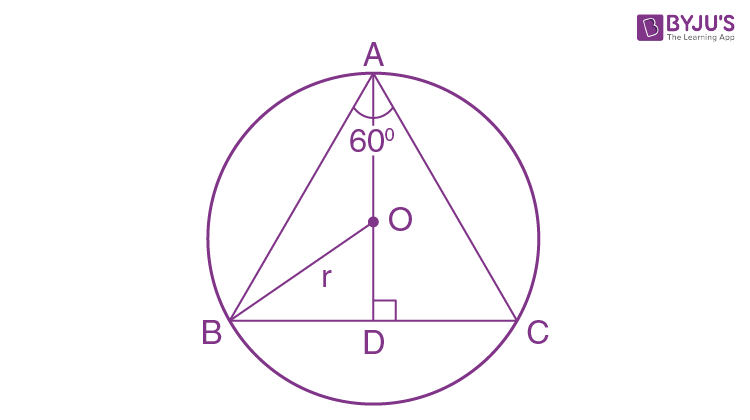Section – B

Question 1: The total number of 4-digit numbers whose greatest common divisor with 18 is 3, is _______

Since the required number has G.C.D with 18 as 3. It must be an odd multiple of ‘3’ but not a multiple of ‘9’.

(i) Now, a 4-digit number which is an odd multiple of ‘3’ are,

1005, 1011, 1017, ? , 9999 → 1499

(ii) The 4-digit number which is an odd multiple of 9 are,

1017, 1035, ? , 9999 → 499

Required numbers = 1499 − 499 = 1000

Question 2: Let ? and β be two real numbers such that ? + β = 1 and ? β = – 1. Let Pn = (?)n + (β)n, Pn-1 = 11 and Pn+1 = 29 for some integer n= 1. Then, the value of Pn2 is______________.

Given, ? + β = 1 and ? β = – 1

A quadratic equation with roots ?, β is x2 – x – 1 = 0.

?2 = ? + 1

Multiplying both sides by ?n-1

?n+1 = ?n + ?n-1 — (1)

Similarly,

βn+1 = βn + βn-1 — (2)

?n+1 + βn+1 = (?)n + (β)n + [?n-1 + βn-1]

Pn+1 = Pn + Pn-1

29 = Pn + 11

Pn = 18

Pn2 = 182 = 324

Question 3: Let X1, X2, …. X18 be eighteen observation such that ∑i=118 (Xi – ?) = 36 and ∑i=118 (Xi – β)2 = 90, where ? and β are distinct real numbers. If the standard deviation of these observations is 1, then the value of |? – β| is ___________.

Given, ∑i=118 (Xi – ?) = 36

∑xi – 18? = 36

∑xi = 18 (? + 2) —- (1)

i=118 (Xi – β)2 = 90

∑xi2 + 18β2 – 2β ∑xi = 90

∑xi2 + 18β2 – 2β * 18 (? + 2) = 90 (using equation (1))

∑xi2 = 90 – 18β2 + 36β (? + 2)

Now, ??2 = 1 ⇒ (1 / 18) ∑xi2 – (∑xi / 18)2 = 1

(1 / 18) [90 – 18β2 + 36?β + 72β] – [18 (? + 2) / 18]2 = 1

5 − β2 + 2?β + 4β − (? + 2)2 = 1

⇒ 5 − β2 + 2?β + 4β − ?2 − 4 − 4? = 1

⇒ ?2 − β2 + 2?β + 4β − 4? = 0

⇒ (? − β)(? − β + 4) = 0

⇒ ? − β = −4

Hence |? − β| = 4 (? ≠ β).

Question 4: In Im,n = ∫01 xm-1 (1 – x)n-1 dx, for m, n ≥ 1 and ∫01 [xm-1 + xn-1] / [(1 + x)m+n] dx = ?Im,n, ? belongs to R, then ? =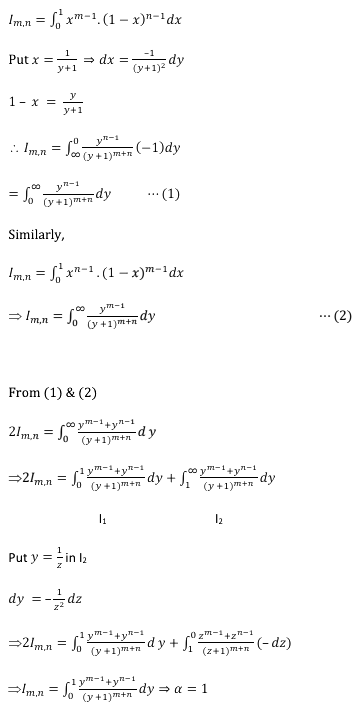Question 5: Let L be a common tangent line to the curves 4x2 + 9y2 = 36 and (2x)2 + (2y)2 = 31. Then the square of the slope of the line L is ______________.

E : (x2 / 9) + (y2 / 4) = 1

C : x2 + y2 = 31 / 4

The equation of the tangent to the ellipse is y = mx ± √(9m2 + 4) — (1)

The equation of the tangent to the circle is y = mx ± √[(31 / 4) m2 + (31 / 4)] — (2)

Comparing equation (1) & (2), 9m2 + 4 = (31 / 4) m2+ (31 / 4)

36m2 + 16 = 31m2 + 31

5m2 = 15

m2 = 3

Question 6: If the matrix

$$\begin{array}{l}A = \begin{bmatrix} 1 & 0 & 0\\ 0 & 2 & 0\\ 3 & 0 & -1 \end{bmatrix}\end{array}$$
satisfies the equation A20 + ?A19 + βA =
$$\begin{array}{l}\begin{bmatrix} 1 & 0 & 0\\ 0 & 4 & 0\\ 0 & 0 & 1 \end{bmatrix}\end{array}$$
for some real numbers ? and β, then β -? is equal to_________.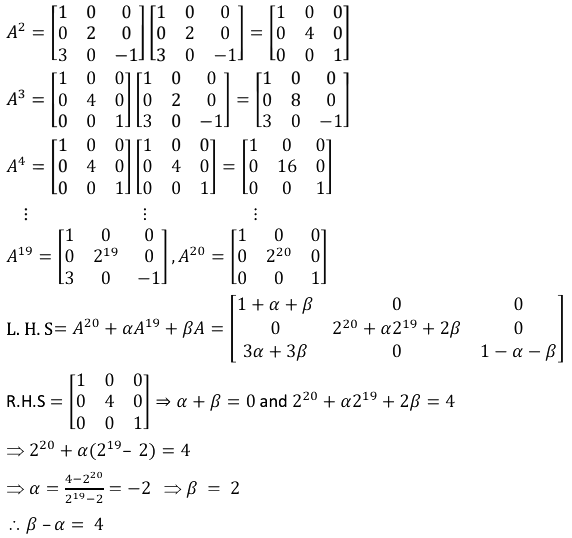Question 7: If the arithmetic mean and the geometric mean of the pth and qth terms of the sequence – 16, 8, – 4, 2, … satisfy the equation 4x2 – 9x + 5 = 0, then p + q is equal to ______________.

Given, 4x2 – 9x + 5 = 0

(x – 1) (4x – 5) = 0

AM = 5 / 4, GM = 1 (AM ≥ GM)

Again for the series, – 16, 8, – 4, 2, …

pth term tp = – 16 (- 1 / 2)p-1

qth term tq = – 16 (- 1 / 2)q-1

AM = [tp + tq] / 2 = 5 / 4

GM = √(tptq) = 1

162 (- 1 / 2)p+q-2 = 1

(- 2)8 = (- 2)p+q-2

p + q = 10

Question 8: Let the normals at all the points on a given curve pass through a fixed point (a, b). If the curve passes through (3, – 3) and (4, – 2 √2), and given that a – 2√2b = 3, then (a2 + b2 + ab) is equal to______________.

Let the equation of normal is Y – y = (- 1 / m) (X – x), where m = dy / dx

As it passes through (a, b)

b – y = (- 1 / m) (a – x) = – dx / dy [a – x]

(b – y) dy = (x – a) dx

by – (y2 / 2) = (x2 / 2) – ax + c — (i)

It passes through (3, – 3) & (4, – 2 √2)

-3b – (9 / 2) = (9 / 2) – 3a + c

3a – 3b – c = 9 — (ii)

Also – 2√2b – 4 = 8 – 4a + c

4a – 2 √2b – c = 12 — (iii)

Also a – 2 √2b = 3 — (iv) (given)

From (ii) – (iii), – a + (2√2 – 3) b = – 3 —- (v)

From (iv) + (v), b = 0, a = 3

a2 + b2 + ab = 9

Question 9: Let z be those complex number which satisfies |z + 5| ≤ 4 and z (1 + i) +

$$\begin{array}{l}\bar{z}\end{array}$$
(1 – i) ≥ – 10, i = √-1. If the maximum value of |z + 1|2 is ? + β√2, then the value of ? + β is ________.

Given, |z + 5| ≤ 4

(x + 5)2 + y2 ≤ 16

Also, z (1 + i) +

$$\begin{array}{l}\bar{z}\end{array}$$
(1 – i) ≥ – 10

x – y ≥ – 5 —- (2)

From (1) and (2), the locus of z is the shaded region in the diagram.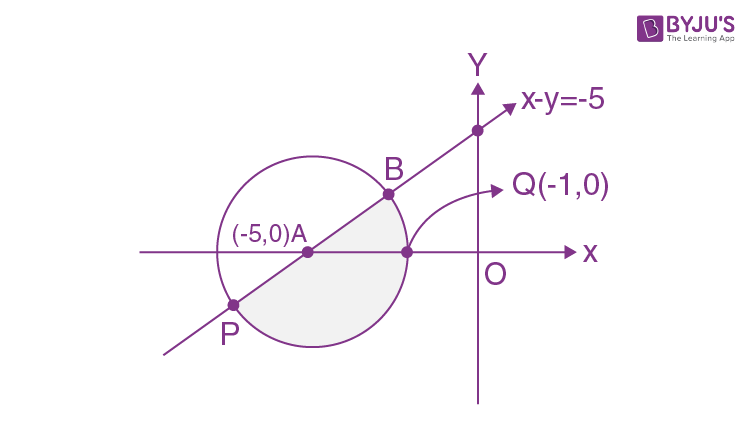|z + 1| represents the distance of ‘z’ from Q (- 1, 0).

Clearly, p is the required position of z when |z + 1| is maximum.

P ≡ (- 5 – 2 √2, – 2 √2)

PQ2 = 32 + 16 √2

? = 32

β = 16

? + β = 48

Question 10: Let a be an integer such that all the real roots of the polynomial 2x5 + 5x4 + 10x3 + 10x2 + 10x + 10 lie in the interval (a, a + 1). Then, |a| is equal to _______.

Let f (x) = 2x5 + 5x4 + 10x3 + 10x2 + 10x + 10

f’ (x) = 10 (x4 + 2x3 + 3x2 + 2x + 1)

= 10x2 [x2 + (1 / x2) + 2 [x + (1 / x)] + 3]

= 10x2 [(x + (1/x))2 + 2 (x + (1 / x)) + 1]

= 10x2 [[x + (1 / x)] + 1]2 > 0, for all x belongs to R

f (x) is strictly increasing function. Since it is an odd degree polynomial it will have exactly one real root.

By observation, f (- 1) = 3 > 0

f (- 2) = – 64 + 80 – 80 + 40 – 20 + 10

= – 34 < 0

f (x) has at least one root in (- 2, – 1) = (a, a + 1)

|a| = 2

#### Video Solutions- February 2021 Question Papers